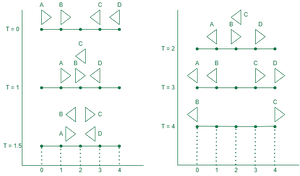Open in App
Not now

# Find the time when last car reaches end of track in given race

• Last Updated : 22 Mar, 2022

Given a car race track of length N units. where each car moves with a speed of 1 unit per second. Given two arrays left[] and right[] denoting the positions of the cars moving towards left and right respectively. When two cars running in two opposite directions meet at some point on the track, they change their directions and continue moving again in reversed directions. Return the time when the last car reaches either of the ends of the track.

Note: No two cars are on the same spot initially.

Examples:

Input: N = 4, left = {4, 3}, right = {0, 1}
Output: 4
Explanation:
The car at index 0 is named A and going to the right.
The car at index 1 is named B and going to the right.
The car at index 3 is named C and going to the left.
The car at index 4 is named D and going to the left.
The last moment when a car was on the race track is t = 4 seconds.
See the image given –Input: N = 7, left = {0, 1, 2, 3, 4, 5, 6, 7}, right = { }
Output: 7
Explanation: All cars are going to the left, the car at index 7 needs 7 seconds to reach the end of track.

Input: N = 10, left = {3, 5}, right = {1}
Output: 9
Explanation:
Say at t = 0, carA = 1 (->), carB = 3 (<-), carC = 5 (<-)
At t = 1: carA and carB collides at 2 and change directions.
So, carA = 2 (<-), carB = 2 (->), carC = 4 (<-)
At t = 2, carB and carC collides at 3 and change direction.
So, carA = 1 (<-), carB = 3 (<-), carC = 3 (->)
At t = 3, carA reaches 0.
So, carA = 0 (<-), carB = 2 (<-), carC = 4 (->)
At t = 4, only carB and carC are running on track in opposite directions.
So, carB = 1 (<-), carC = 5 (->)
At t = 5, carB reaches at 0.
So, carB = 0 (<-), carC = 5 (->)
carC alone running towards right end (index 9).
So, at t = 9 carC reaches at 9.

Approach: The problem can be solved based on the following observation.
Since the cars on collisions change directions immediately and start moving in the opposite direction, it can be considered as the colliding cars are just swapping their position and moving in the same direction afterward. So the required time is the maximum time any car takes to reach from the starting position to the end if it keeps on moving in the same direction.

Below is the implementation of the above approach.

## C++

 `// Program for above approach.` `#include ` `using` `namespace` `std;`   `// Function to get the maximum time` `int` `getLastCarMoment(``int` `N,` `                     ``vector<``int``>& left,` `                     ``vector<``int``>& right)` `{` `    ``int` `ans = 0;` `    ``for` `(``auto` `it : left)` `        ``ans = max(ans, it);`   `    ``for` `(``auto` `it : right)` `        ``ans = max(ans, N - it);`   `    ``return` `ans;` `}`   `// Driver code` `int` `main()` `{` `    ``int` `N = 10;` `    ``vector<``int``> left{ 3, 5 }, right{ 1 };`   `    ``// Function call` `    ``int` `res = getLastCarMoment(N, left, right);` `    ``cout << res;` `    ``return` `0;` `}`

## Java

 `// Java Program for above approach.` `class` `GFG {`   `    ``// Function to get the maximum time` `    ``static` `int` `getLastCarMoment(``int` `N,` `            ``int``[] left,` `            ``int``[] right) {` `        ``int` `ans = ``0``;` `        ``for` `(``int` `it : left)` `            ``ans = Math.max(ans, it);`   `        ``for` `(``int` `it : right)` `            ``ans = Math.max(ans, N - it);`   `        ``return` `ans;` `    ``}`   `    ``// Driver code` `    ``public` `static` `void` `main(String args[]) {` `        ``int` `N = ``10``;` `        ``int``[] left = { ``3``, ``5` `};` `        ``int``[] right = { ``1` `};`   `        ``// Function call` `        ``int` `res = getLastCarMoment(N, left, right);` `        ``System.out.println(res);` `    ``}` `}`   `// This code is contributed by Saurabh jaiswal`

## Python3

 `# Program for above approach.`   `# Function to get the maximum time` `def` `getLastCarMoment(N,` `                     ``left,` `                     ``right):`   `    ``ans ``=` `0` `    ``for` `it ``in` `left:` `        ``ans ``=` `max``(ans, it)`   `    ``for` `it ``in` `right:` `        ``ans ``=` `max``(ans, N ``-` `it)`   `    ``return` `ans`   `# Driver code` `if` `__name__ ``=``=` `"__main__"``:`   `    ``N ``=` `10` `    ``left ``=` `[``3``, ``5``]` `    ``right ``=` `[``1``]`   `    ``# Function call` `    ``res ``=` `getLastCarMoment(N, left, right)` `    ``print``(res)`   `    ``# This code is contributed by ukasp.`

## C#

 `// Program for above approach.` `using` `System;` `class` `GFG` `{`   `  ``// Function to get the maximum time` `  ``static` `int` `getLastCarMoment(``int` `N,` `                              ``int` `[]left,` `                              ``int` `[]right)` `  ``{` `    ``int` `ans = 0;` `    ``foreach` `(``int` `it ``in` `left)` `      ``ans = Math.Max(ans, it);`   `    ``foreach` `(``int` `it ``in` `right)` `      ``ans = Math.Max(ans, N - it);`   `    ``return` `ans;` `  ``}`   `  ``// Driver code` `  ``public` `static` `void` `Main()` `  ``{` `    ``int` `N = 10;` `    ``int` `[]left = { 3, 5 };` `    ``int` `[]right = { 1 };`   `    ``// Function call` `    ``int` `res = getLastCarMoment(N, left, right);` `    ``Console.Write(res);` `  ``}` `}`   `// This code is contributed by Samim Hossain Mondal.`

## Javascript

 ``

Output

`9`

Time Complexity: O(M) where M is the maximum size of the two arrays.
Auxiliary Space: O(1)

My Personal Notes arrow_drop_up
Related Articles